【一猫汽车网】“现代广告之父”于勒·谢雷(Jules Chéret)于19世纪与20世纪之际在巴黎绘制出第一张大幅广告，宣告了现代广告业的开端。

【一猫汽车网】“现代广告之父”于勒·谢雷(Jules Chéret)于19世纪与20世纪之际在巴黎绘制出第一张大幅广告，宣告了现代广告业的开端。作为世界五大广告国之一，法国广告业的发达与其悠久的历史、丰富的自然人文景观不无关系。香水、时装、美食、旅游、历史等等，都是法国广告宣传的内容。而作为现代社会标志之一的汽车，自然是广告不会错过的主体。百年历程，法国汽车制造商雷诺，用一幅幅精心设计的广告在助力营销的同时，也展示了跨越一个多世纪的法国社会生活史和变迁史。

1898年，雷诺用另一种形式的“广告”让世人瞩目。创始人路易·雷诺（LouisRenault）驾驶着自行研发的Type A在巴黎蒙马特攀上了陡斜的勒比克街。他用行动为公司赢得了首批订单，也为“雷诺”打出了第一个响亮的广告。

在20世纪早期的雷诺广告中，使用了复古的字体与绘画，凸显速度与空气动力学设计，反映出鲜明的时代特征。雷诺广告试图展示法国人的声望、专业性以及当时取得巨大成就的另一个主要领域：航空。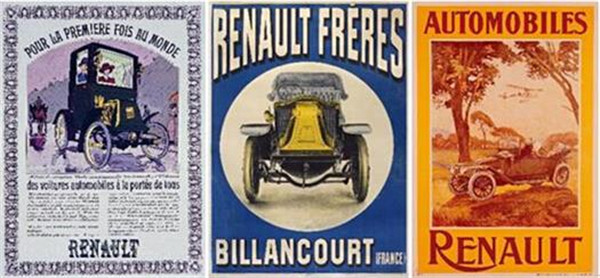二战后，雷诺被收为国有，法国社会也经历了众多变化。婴儿潮、城郊发展以及妇女解放运动，为雷诺带来了新的消费者。1946年，雷诺推出了4CV，这是标志着法国战后繁荣年代开始的一款车型，也是雷诺第一款销量过百万的车型。著名插图画家雷蒙德·萨维尼亚克（RaymondSavignac）特意为雷诺创作海报来赞颂4CV。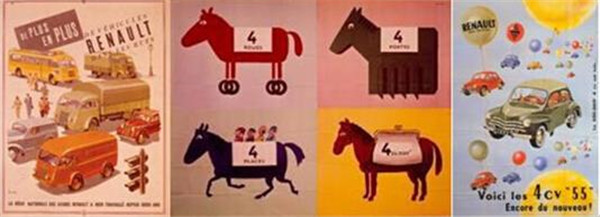20世纪60年代开始，雷诺在广告中不再采用绘画，而是采用照片形式来展现车辆。雷诺4在1961年的首次宣传中突出燃油经济性的特色；1963年的宣传则更强调Parisienne车型的女性气质。十年之后，著名歌手米歇尔•弗甘（MichelFugain）还在歌唱雷诺4：“faisuntourenRenault4,demande-luibeaucoup（开着雷诺4去兜风，它绝不会让你失望）”。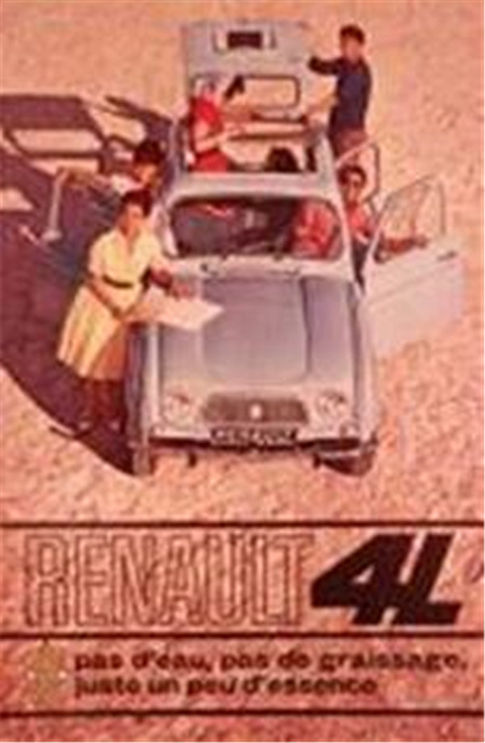1972年，雷诺的广告口号变得更加贴近生活——“雷诺，生活之车”（Renault, Des Voitures à Vivre）。“生活之车”进一步确立了雷诺满足男性、女性和家庭实用与精神需求的特点：在法国人生活中的时时刻刻，都有雷诺陪伴左右。同年，雷诺发布了面向年轻消费者的雷诺5。这次广告利用代表当代年轻人喜好的轮滑，突出了雷诺5的动感特质，也展现了年轻一代向往自由的精神。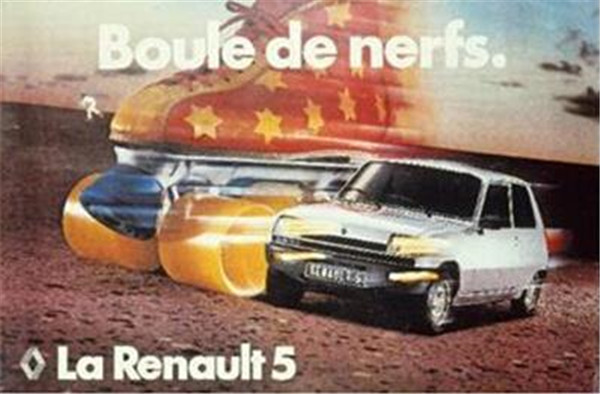20世纪80年代，全球陷入不安与动荡。此时的雷诺4打出了“Ellesupermarchebien”（她是超市货）的口号，强调产品性价比——“bien marcher”（性能优异）和“supermarché”（超市货）。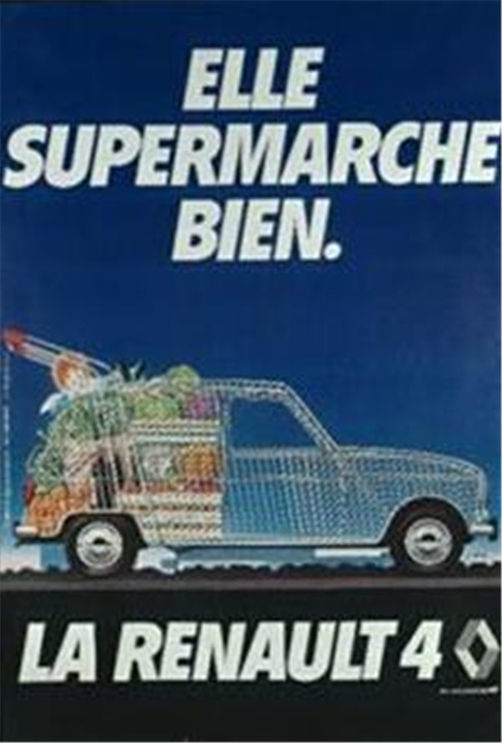20世纪90年代，雷诺通过推出Twingo和Clio两款车型重新定义了小型车。在广告中，五颜六色的单厢小型车Twingo圆滑可爱，倡导消费者“创造与车同行的生活方式”，而Clio则使众人都能享受到汽车的高品质。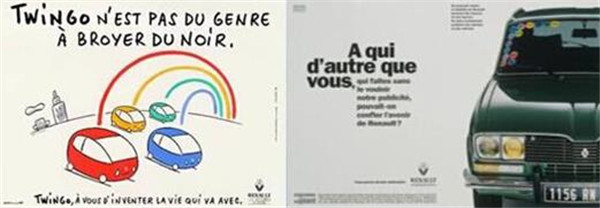进入21世纪，雷诺启用了全新的设计方案，让汽车设计回归本质，提出了以“人生阶段”（Cycle of Life）为主线的全新设计理念，于2012年发布新版Clio。新版Clio以其火红色的车身带给车迷感官上的刺激，重燃世界各地雷诺车迷心中的火焰。2014年，全新Twingo发售在即，雷诺邀请视觉艺术家Kuntzel + Deygas创作了一支美貌活跃的小动画。Twingo跨过了缤纷季节，穿过神秘国度，甚至乱入了一下拍片现场。这支动画为爱闯爱拼的年轻人量身打造，去任何地方，到处都去，过不一样的生活，看见不一样的世界。东奔西走的力量与速度，就包在小Twingo身上好了！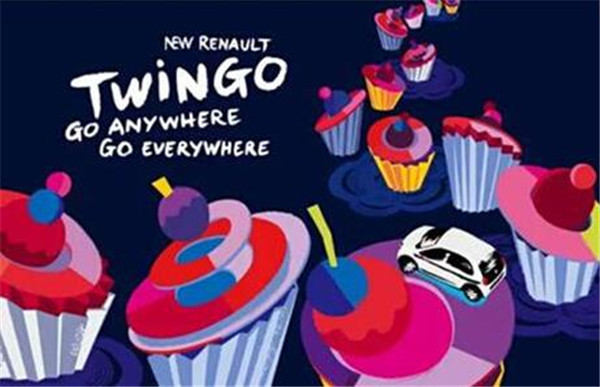2015年，雷诺邀请到国际偶像凯文·史派西（Kevin Spacey）出任雷诺Espace形象代言人。从遭遇中年危机的父亲到声名狼藉的瘸子、再到美国总统，凯文·史派西一直在扮演着别人，而当他手握新版雷诺Espace的方向盘时，凯文终于扮演了一回自己，在驾驶中享受时间的流逝……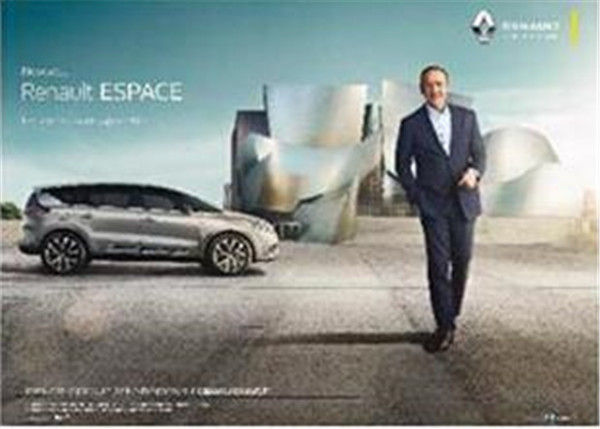百年来，雷诺广告将风趣又不失深邃的视角投向法国和法兰西人民的生活，折射出法国人的内心世界。透过这些广告，我们得以艳遇法国的浪漫与璀璨，并窥见雷诺品牌的历史与激情。

|分享

•已阅！握爪
•我手滑为你点赞
•128个赞！
•不明觉厉
•阅后既醉
•有钱！任性
•照片太美，我不敢看
•干货！杠杠的
•高大上
•膜拜中！
•请接受我的膝盖
•猫哥/猫妹么么哒
•神吐槽
•我只笑笑不说话
•我想静静
•窒息
##### 热度排行• 阿斯顿·马丁
• 奥迪
• 阿尔法罗密欧
• ALPINA

• 巴博斯
• 宝骏
• 宝马
• 保时捷
• 北汽制造
• 奔驰
• 奔腾
• 本田
• 比亚迪
• 标致
• 别克
• 宾利
• 布加迪
• 北汽威旺
• 北京
• 北汽绅宝
• 北汽幻速
• 北汽新能源
• 宝沃
• 比速汽车
• 北汽道达

• 昌河
• 长安
• 长城
• 长安商用
• 成功汽车
• 长江EV
• 长安轻车型

• 大众
• 道奇
• 东风
• 东风风神
• 东风小康
• 东南
• DS
• 东风风行
• 东风风度
• 东风风光

• 法拉利
• 菲亚特
• 丰田
• 福迪
• 福特
• 福田汽车
• 福汽启腾
• 风诺

• GMC
• 广汽传祺
• 广汽吉奥
• 观致

• 哈飞
• 海格
• 海马
• 华泰
• 黄海
• 恒天
• 红旗
• 哈弗
• 华颂
• 华凯
• 华泰新能源
• 汉腾汽车

• Jeep
• 江淮
• 江铃
• 捷豹
• 金杯
• 九龙
• 吉利汽车
• 金旅
• 金龙
• 江铃集团轻汽
• 江铃集团新能源
• 君马汽车
• 捷途

• 开瑞
• 凯迪拉克
• 科尼赛克
• 克莱斯勒
• KTM
• 卡威
• 凯翼
• 康迪
• 康迪电动汽车集团

• 猎豹汽车
• 兰博基尼
• 劳斯莱斯
• 雷克萨斯
• 雷诺
• 理念
• 力帆
• 莲花汽车
• 林肯
• 铃木
• 陆风
• 路虎
• 路特斯
• 领志
• 领克
• 零跑汽车

• MG
• MINI
• 马自达
• 玛莎拉蒂
• 迈凯伦
• 摩根

• 纳智捷

• 讴歌
• 欧宝
• 欧朗
• 欧拉
• 欧尚汽车

• 帕加尼

• 奇瑞
• 启辰
• 起亚
• 前途
• 庆铃汽车

• 日产
• 荣威
• 瑞麒汽车
• 如虎
• 瑞驰

• 上汽大通
• smart
• 三菱
• 双环
• 双龙
• 斯巴鲁
• 斯柯达
• 萨博
• 思铭
• 赛麟
• SWM斯威汽车

• TESLA
• 泰卡特
• 腾势

• 威麟
• 威兹曼
• 沃尔沃
• 五菱汽车
• 五十铃
• 潍柴英致
• WEY
• 蔚来
• 威马汽车

• 现代
• 雪佛兰
• 雪铁龙
• 西雅特
• 新特汽车
• 小鹏汽车
• 新宝骏

• 野马汽车
• 一汽
• 依维柯
• 英菲尼迪
• 永源
• 驭胜

• 中华
• 中兴
• 众泰
• 知豆
• 之诺
• 正道汽车
• A
• B
• C
• D
• E
• F
• G
• H
• I
• J
• K
• L
• M
• N
• O
• P
• Q
• R
• S
• T
• U
• V
• W
• X
• Y
• Z

• 阿斯顿·马丁
• 奥迪
• 阿尔法罗密欧
• ALPINA

• 巴博斯
• 宝骏
• 宝马
• 保时捷
• 北汽制造
• 奔驰
• 奔腾
• 本田
• 比亚迪
• 标致
• 别克
• 宾利
• 布加迪
• 北汽威旺
• 北京
• 北汽绅宝
• 北汽幻速
• 北汽新能源
• 宝沃
• 比速汽车
• 北汽道达

• 昌河
• 长安
• 长城
• 长安商用
• 成功汽车
• 长江EV
• 长安轻车型

• 大众
• 道奇
• 东风
• 东风风神
• 东风小康
• 东南
• DS
• 东风风行
• 东风风度
• 东风风光

• 法拉利
• 菲亚特
• 丰田
• 福迪
• 福特
• 福田汽车
• 福汽启腾
• 风诺

• GMC
• 广汽传祺
• 广汽吉奥
• 观致

• 哈飞
• 海格
• 海马
• 华泰
• 黄海
• 恒天
• 红旗
• 哈弗
• 华颂
• 华凯
• 华泰新能源
• 汉腾汽车

• Jeep
• 江淮
• 江铃
• 捷豹
• 金杯
• 九龙
• 吉利汽车
• 金旅
• 金龙
• 江铃集团轻汽
• 江铃集团新能源
• 君马汽车
• 捷途

• 开瑞
• 凯迪拉克
• 科尼赛克
• 克莱斯勒
• KTM
• 卡威
• 凯翼
• 康迪
• 康迪电动汽车集团

• 猎豹汽车
• 兰博基尼
• 劳斯莱斯
• 雷克萨斯
• 雷诺
• 理念
• 力帆
• 莲花汽车
• 林肯
• 铃木
• 陆风
• 路虎
• 路特斯
• 领志
• 领克
• 零跑汽车

• MG
• MINI
• 马自达
• 玛莎拉蒂
• 迈凯伦
• 摩根

• 纳智捷

• 讴歌
• 欧宝
• 欧朗
• 欧拉
• 欧尚汽车

• 帕加尼

• 奇瑞
• 启辰
• 起亚
• 前途
• 庆铃汽车

• 日产
• 荣威
• 瑞麒汽车
• 如虎
• 瑞驰

• 上汽大通
• smart
• 三菱
• 双环
• 双龙
• 斯巴鲁
• 斯柯达
• 萨博
• 思铭
• 赛麟
• SWM斯威汽车

• TESLA
• 泰卡特
• 腾势

• 威麟
• 威兹曼
• 沃尔沃
• 五菱汽车
• 五十铃
• 潍柴英致
• WEY
• 蔚来
• 威马汽车

• 现代
• 雪佛兰
• 雪铁龙
• 西雅特
• 新特汽车
• 小鹏汽车
• 新宝骏

• 野马汽车
• 一汽
• 依维柯
• 英菲尼迪
• 永源
• 驭胜

• 中华
• 中兴
• 众泰
• 知豆
• 之诺
• 正道汽车
• A
• B
• C
• D
• E
• F
• G
• H
• I
• J
• K
• L
• M
• N
• O
• P
• Q
• R
• S
• T
• U
• V
• W
• X
• Y
• Z

• 阿斯顿·马丁
• 奥迪
• 阿尔法罗密欧
• ALPINA

• 巴博斯
• 宝骏
• 宝马
• 保时捷
• 北汽制造
• 奔驰
• 奔腾
• 本田
• 比亚迪
• 标致
• 别克
• 宾利
• 布加迪
• 北汽威旺
• 北京
• 北汽绅宝
• 北汽幻速
• 北汽新能源
• 宝沃
• 比速汽车
• 北汽道达

• 昌河
• 长安
• 长城
• 长安商用
• 成功汽车
• 长江EV
• 长安轻车型

• 大众
• 道奇
• 东风
• 东风风神
• 东风小康
• 东南
• DS
• 东风风行
• 东风风度
• 东风风光

• 法拉利
• 菲亚特
• 丰田
• 福迪
• 福特
• 福田汽车
• 福汽启腾
• 风诺

• GMC
• 广汽传祺
• 广汽吉奥
• 观致

• 哈飞
• 海格
• 海马
• 华泰
• 黄海
• 恒天
• 红旗
• 哈弗
• 华颂
• 华凯
• 华泰新能源
• 汉腾汽车

• Jeep
• 江淮
• 江铃
• 捷豹
• 金杯
• 九龙
• 吉利汽车
• 金旅
• 金龙
• 江铃集团轻汽
• 江铃集团新能源
• 君马汽车
• 捷途

• 开瑞
• 凯迪拉克
• 科尼赛克
• 克莱斯勒
• KTM
• 卡威
• 凯翼
• 康迪
• 康迪电动汽车集团

• 猎豹汽车
• 兰博基尼
• 劳斯莱斯
• 雷克萨斯
• 雷诺
• 理念
• 力帆
• 莲花汽车
• 林肯
• 铃木
• 陆风
• 路虎
• 路特斯
• 领志
• 领克
• 零跑汽车

• MG
• MINI
• 马自达
• 玛莎拉蒂
• 迈凯伦
• 摩根

• 纳智捷

• 讴歌
• 欧宝
• 欧朗
• 欧拉
• 欧尚汽车

• 帕加尼

• 奇瑞
• 启辰
• 起亚
• 前途
• 庆铃汽车

• 日产
• 荣威
• 瑞麒汽车
• 如虎
• 瑞驰

• 上汽大通
• smart
• 三菱
• 双环
• 双龙
• 斯巴鲁
• 斯柯达
• 萨博
• 思铭
• 赛麟
• SWM斯威汽车

• TESLA
• 泰卡特
• 腾势

• 威麟
• 威兹曼
• 沃尔沃
• 五菱汽车
• 五十铃
• 潍柴英致
• WEY
• 蔚来
• 威马汽车

• 现代
• 雪佛兰
• 雪铁龙
• 西雅特
• 新特汽车
• 小鹏汽车
• 新宝骏

• 野马汽车
• 一汽
• 依维柯
• 英菲尼迪
• 永源
• 驭胜

• 中华
• 中兴
• 众泰
• 知豆
• 之诺
• 正道汽车
• A
• B
• C
• D
• E
• F
• G
• H
• I
• J
• K
• L
• M
• N
• O
• P
• Q
• R
• S
• T
• U
• V
• W
• X
• Y
• Z